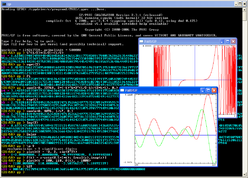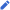# PARI/GP

Developer(s)PARI/GP in use on Windows XP Henri Cohen, Karim Belabas, et al., at the Université Bordeaux 1 2.13.1 / 25 January 2021; 4 months ago pari.math.u-bordeaux.fr/git/pari.gitC Cross-platform Computer algebra system GNU General Public License pari.math.u-bordeaux.fr

PARI/GP is a computer algebra system with the main aim of facilitating number theory computations. Versions 2.1.0 and higher are distributed under the GNU General Public License. It runs on most common operating systems.

## System overview

The PARI/GP system is a package that is capable of doing formal computations on recursive types at high speed; it is primarily aimed at number theorists. Its three main strengths are its speed, the possibility of directly using data types that are familiar to mathematicians, and its extensive algebraic number theory module.

The PARI/GP system consists of the following standard components:

• PARI is a C library, allowing for fast computations, and which can be called from a high-level language application (for instance, written in C, C++, Pascal, Fortran, Perl, or Python).
• gp is an easy-to-use interactive command line interface giving access to the PARI functions. It functions as a sophisticated programmable calculator which contains most of the control instructions of a standard language like C. GP is the name of gp's scripting language which can be used to program gp.

Also available is gp2c, the GP-to-C compiler, which compiles GP scripts into the C language and transparently loads the resulting functions into gp. The advantage of this is that gp2c-compiled scripts will typically run three to four times faster. gp2c understands almost all of GP.

PARI/GP performs arbitrary precision calculations (e.g., the significand can be millions of digits long—and billions of digits on 64-bit machines). It can compute factorizations, perform elliptic curve computations and perform algebraic number theory calculations. It also allows computations with matrices, polynomials, power series, algebraic numbers and implements many special functions.

PARI/GP comes with its own built-in graphical plotting capability. PARI/GP has some symbolic manipulation capability, e.g., multivariate polynomial and rational function handling. It also has some formal integration and differentiation capabilities.

PARI/GP can be compiled with GMP (GNU Multiple Precision Arithmetic Library) providing faster computations than PARI/GP's native arbitrary-precision kernel.

## History

PARI/GP's progenitor was a program named Isabelle, an interpreter for higher arithmetic, written in 1979 by Henri Cohen and François Dress at the Université Bordeaux 1.

PARI/GP was originally developed in 1985 by a team led by Henri Cohen at Laboratoire A2X and is now maintained by Karim Belabas at the Université Bordeaux 1 with the help of many volunteer contributors.

### Etymology

The name PARI is a pun about the project's early stages when the authors started to implement a library for "Pascal ARIthmetic" in the Pascal programming language (although they quickly switched to C), and after "pari de Pascal" (Pascal's Wager).

The first version of the gp calculator was originally called GPC, for Great Programmable Calculator. The trailing C was eventually dropped.

## Usage examples

Below are some samples of the gp calculator usage:

```? \p 212
realprecision = 221 significant digits (212 digits displayed)
? (1.378-0.09143*I)^(14.87+0.3721*I)
time = 0 ms.
%1 = 80.817082637557070449383034933010288336925078193546211741027496566803185
11092579265743992920628314516739962724446042667886245322716456966120413965187
3272488827365261487845201056199035423784093096984005713791800191 - 94.8384618
89186304973351271821601500916571303364865064205039706592481303045713982306764
33264430511752515705768858710051382035377195497482934017239179757538824688799
0680136241031895212412150770309289450962931402933*I

? 123456! + 0.    \\ slower than gamma(123457) which uses floating point
time = 1,656 ms.
%2 = 2.6040699049291378729513930560926568818273270409503019584610185579952057
37967683415793560716617127908735520017061666000857261271456698589373086528293
4317244121152865814030204645985573419251305342231135573491050756 E574964

? sin(x)
time = 0 ms.
%3 = x - 1/6*x^3 + 1/120*x^5 - 1/5040*x^7 + 1/362880*x^9 - 1/39916800*x^11
+ 1/6227020800*x^13 - 1/1307674368000*x^15 + O(x^17)

? for(z=25,30, print (factor(2^z-1)))
[31, 1; 601, 1; 1801, 1]
[3, 1; 2731, 1; 8191, 1]
[7, 1; 73, 1; 262657, 1]
[3, 1; 5, 1; 29, 1; 43, 1; 113, 1; 127, 1]
[233, 1; 1103, 1; 2089, 1]
[3, 2; 7, 1; 11, 1; 31, 1; 151, 1; 331, 1]
time = 5 ms.

? K = bnfinit(x^2 + 23); K.cyc
time = 1ms.
%4 = 
/* This number field has class number 3. */
```